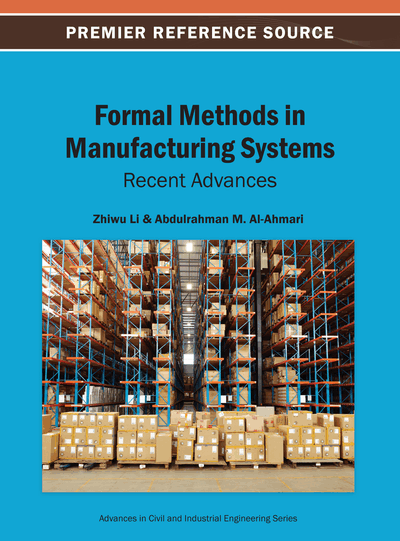# Hybrid Optimization Techniques for Industrial Production Planning

Pandian Vasant (Universiti Teknologi PETRONAS, Malaysia)
DOI: 10.4018/978-1-4666-4034-4.ch005
Available
\$33.75
List Price: \$37.50
10% Discount:-\$3.75
TOTAL SAVINGS: \$3.75

## Abstract

In this chapter, the main significant contributions of a new non-linear membership function using fuzzy approach to capture and describe vagueness in the technological coefficients of constraints in the industrial production planning problems are investigated thoroughly. This non-linear membership function is flexible and convenient to the decision makers in their decision making process. Secondly, a nonlinear objective function in the form of cubic function for fuzzy optimization problems is successfully solved by 15 hybrid and non-hybrid optimization techniques from the area of soft computing and classical approaches. Among the 15 techniques, three outstanding techniques are selected based on the percentage of quality solution. An intelligent performance analysis table is tabulated to the convenience of decision makers and implementers to select the niche optimization techniques to apply in real word problem solving approach particularly related to industrial engineering problems.
Chapter Preview
Top

## Introduction

In general, an optimization problem requires finding a settingof free parameters of the system under consideration, such that a certain criterion(usually called the objective function) is maximized (or, minimized) (Back, 1996)

(1)

The objective function might be given by real-world systems of arbitrary complexity. The solution to the global optimization problem (1) requires finding a vectorsuch thatCharacteristic such as multimodality, the existence of several local maximaif

(2) (Wheredenotes a distance measure on M), constraints, restrictions on the set M by functionsuch that the set of feasible solutionsis only subset of the domain of the variables (3) And other factors, such as large dimensionality, strong non-linearities, nondifferentiability, and noisy and time-varying objective functions, frequently lead to difficulties if not solvable optimization tasks (Torn and Zilinskas, 1989). But even in the latter case, the identification of an improvement of the currently known best solution through optimization is often already a big success for practical problems, and in many cases evolutionary algorithms provide an efficient and effective method to achieve this objective.

Optimization problems occur in many engineering, economics, and scientific research projects, like cost, time, and risk minimization or quality, revenue, and efficiency maximization (Back, Fogel and Michalewicz, 1997). Thus, the development of general strategies is of great value.

In real-world situations, the objective function f and the constraints gj are often not analytically treatable or are even not given in closed form, and the function definitions are based on a simulation model (Schwefel, 1979).

The traditional approach in such a case is to develop a formal model that resembles the original functions close enough but is solvable by means of traditional mathematical methods such as linear and non-linear programming. This approach most often requires simplifications of the original problem formulation. Thus, an important aspect of mathematical programming lies in the design of the formal model.

No doubt, this approach has proven to be very successful in many applications, but has several drawbacks, which motivated the search for novel approaches, where evolutionary computation is one of the most promising directions. The most severe problem is that, due to over simplifications; the computed solutions do not solve the original problem. Such problems, in the case of simulation models, are often considered as unsolvable.

## Complete Chapter List

Search this Book:
Reset